# A Beginner's Guide to Machine Learning: What Aspiring Data Scientists Should Know

### Learn all about machine learning and it's different subsets, such as supervised learning, unsupervised learning, and the subsets within those tops.

· AI Zone · Opinion
Save
14.23K ViewsBefore choosing a machine learning algorithm, it's important to know their characteristics to generate desired outputs and build smart systems.

Data science is growing super fast. As the demand for AI-enabled solutions is increasing, delivering smarter systems for industries has become essential. And the correctness and efficiency through machine learning operations must be fulfilled to ensure the developed solutions complete all demands. Hence, applying machine learning algorithms on the given dataset to produce righteous results and train the intelligent system is one of the most essential steps from the entire process.

## Machine Learning: Training for Better Results and Great Achievements

Machine learning is merely an application of AI that makes a machine learn automatically and evolve according to the user requirements to fulfill every demand they bring out. The concepts of machine learning are easy to understand, while the implementation is complex and requires expert support. It consists of the programs developed to access the data stored on the system and make it more intelligent by studying the patterns and providing better support through continuous learning.

You may also like:  10 Machine Learning Algorithms You Should Know to Become a Data Scientist

The gathered data is processed via different methods and algorithms of machine learning developed to predict outcomes from it. However, the algorithm changes according to the requirements specified. A single algorithm cannot fulfill every purpose, therefore, choosing the right algorithm according to the learning methods is required.

## What Do Machine Learning Algorithms and Processes Provide as Output?

The outputs from machine learning processes are the information gained from the existing data. It simply extracts the knowledge it can gather from the raw data and develops the ability to predict the next outcomes by learning patterns and possible chances of an entity or event occurrences. The practical applications of machine learning can be listed as:

### 1. Prediction

After processing the existing data, the machine or system can be enabled to predict the next outcomes from the provided details. For example, weather forecasting.

### 2. Image and Speech Recognition

Studying the data from each pixel of provided images and characteristics from the voice or image, the provided input can be identified and classified by the knowledge developed from existing data.

By observing the patterns from the existing data, machine learning algorithms can predict and tell whether investments are profitable or not and guide on the right path to prevent losses. For example, an AI-driven hedge fund is implemented for gaining the best results using machine learning and AI.

### 4. Medical Diagnosis

When provided with complete data and possible outcomes for each entry, the system predicts the possibilities of disease occurrences in a particular person using machine learning algorithms.

There are primary applications where machine learning algorithms provide extended support for predicting or help develop knowledge that can generate correct outputs by further processing.

## Types of Machine Learning Algorithms

Machine learning can be divided into three types:

### 1. Supervised Learning

In supervised learning, the data fed to the system is labeled. Here, labeled data is defined as the data that has been divided into the righteous groups and the machine can understand that each entry is different from any other belonging to other classes. Hence, using supervised learning, the machine is provided with data that has already been classified and datasets are created.

Using supervised learning, the aim is to develop a mapping function that when applied to the input data can predict certain outcomes. Many times, a single instance from the data is used repetitively to check whether the implemented model is working or not. There are 2 types of algorithms covered under supervised learning:

#### 1. 1 Classification

A classification model will predict the category of given input data from the existing data by classifying according to the parameter values. The classification does not provide values as output, instead, it categorizes the data using the existing data in provided classes.

##### Logistic Regression

The most simple example of classification is logistic regression. Based on the input data, classification can be of binary classes or multi-classes, and logistic regression is an example of binary classification, which the input data can be divided (in 0/1, true/false or more categories) i.e., based on the input data, it classifies the data in up to two distinct classes.  It predicts the output value in terms of probability, which lies between 0 and 1. Also called logit regression, this classification algorithm classifies the input data in terms of the probability. It can be represented simply as:

`````` odds= pr/ (1-pr) = probability of occurrence / probability of not event occurrence
loge(odds) = loge(pr/(1-pr))
logit(pr) = ln(pr/(1-pr)) = b0+b1X1+b2X2+b3X3....+bkXk ``````

Here, “pr” represents the probability of a characteristic present in the input. It simply works by selecting the right parameters of the input that increase the likelihood of event occurrence.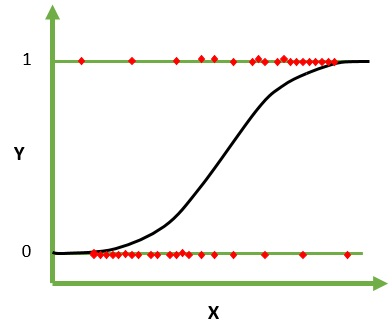Logistic Regression (source)

Here is the R code of an example implementing logistic regression that you can run on your operating system if R is already installed. And if not, learn how to install R for your operating system here.

``````x <- cbind(x_train,y_train)
# Train the model using the training sets and check score
logistic <- glm(y_train ~ ., data = x,family='binomial')
summary(logistic)
#Predict Output
predicted= predict(logistic,x_test)``````

Alternatively, you can use Python to run and observe the outputs provided. The python code for the same example is:

``````# importing required libraries
import pandas as pd
from sklearn.linear_model import LinearRegression
from sklearn.metrics import mean_squared_error

# read the train and test dataset

# shape of the dataset
print('\nShape of training data :',train_data.shape)
print('\nShape of testing data :',test_data.shape)

# Now, we need to predict the missing target variable in the test data
# target variable - Item_Outlet_Sales

# seperate the independent and target variable on training data
train_x = train_data.drop(columns=['Item_Outlet_Sales'],axis=1)
train_y = train_data['Item_Outlet_Sales']

# seperate the independent and target variable on training data
test_x = test_data.drop(columns=['Item_Outlet_Sales'],axis=1)
test_y = test_data['Item_Outlet_Sales']

'''
Create the object of the Linear Regression model
Some parameters are : fit_intercept and normalize
Documentation of sklearn LinearRegression:

https://scikit-learn.org/stable/modules/generated/sklearn.linear_model.LinearRegression.html

'''
model = LinearRegression()

# fit the model with the training data
model.fit(train_x,train_y)

# coefficeints of the trained model
print('\nCoefficient of model :', model.coef_)

# intercept of the model
print('\nIntercept of model',model.intercept_)

# predict the target on the test dataset
predict_train = model.predict(train_x)
print('\nItem_Outlet_Sales on training data',predict_train)

# Root Mean Squared Error on training dataset
rmse_train = mean_squared_error(train_y,predict_train)**(0.5)
print('\nRMSE on train dataset : ', rmse_train)

# predict the target on the testing dataset
predict_test = model.predict(test_x)
print('\nItem_Outlet_Sales on test data',predict_test)

# Root Mean Squared Error on testing dataset
rmse_test = mean_squared_error(test_y,predict_test)**(0.5)
print('\nRMSE on test dataset : ', rmse_test)
``````

#### Decision Tree

A decision tree algorithm classifies the data in categories by making homogeneous classes of existing data and significant parameters that lead the entry in the right category. A decision tree algorithm can be used for both categorical or independent variables to improve the efficiency of predicting.

Below is an example of a decision tree algorithm implementation using Python on the dataset Balance Scale Weight and Distance. The algorithm effectively classifies the entities, and the accuracy of it can be found using different measures like the Gini index, entropy, and more.

``````# Importing the required packages
import numpy as np
import pandas as pd
from sklearn.metrics import confusion_matrix
from sklearn.cross_validation import train_test_split
from sklearn.tree import DecisionTreeClassifier
from sklearn.metrics import accuracy_score
from sklearn.metrics import classification_report

# Function importing Dataset
def importdata():
'https://archive.ics.uci.edu/ml/machine-learning-'+
'databases/balance-scale/balance-scale.data',

# Printing the dataset shape
print ("Dataset Length: ", len(balance_data))
print ("Dataset Shape: ", balance_data.shape)

# Printing the dataset observations
return balance_data

# Function to split the dataset
def splitdataset(balance_data):

# Separating the target variable
X = balance_data.values[:, 1:5]
Y = balance_data.values[:, 0]

# Splitting the dataset into train and test
X_train, X_test, y_train, y_test = train_test_split(
X, Y, test_size = 0.3, random_state = 100)

return X, Y, X_train, X_test, y_train, y_test

# Function to perform training with giniIndex.
def train_using_gini(X_train, X_test, y_train):

# Creating the classifier object
clf_gini = DecisionTreeClassifier(criterion = "gini",
random_state = 100,max_depth=3, min_samples_leaf=5)

# Performing training
clf_gini.fit(X_train, y_train)
return clf_gini

# Function to perform training with entropy.
def tarin_using_entropy(X_train, X_test, y_train):

# Decision tree with entropy
clf_entropy = DecisionTreeClassifier(
criterion = "entropy", random_state = 100,
max_depth = 3, min_samples_leaf = 5)

# Performing training
clf_entropy.fit(X_train, y_train)
return clf_entropy

# Function to make predictions
def prediction(X_test, clf_object):

# Prediction on test with giniIndex
y_pred = clf_object.predict(X_test)
print("Predicted values:")
print(y_pred)
return y_pred

# Function to calculate accuracy
def cal_accuracy(y_test, y_pred):

print("Confusion Matrix: ",
confusion_matrix(y_test, y_pred))

print ("Accuracy : ",
accuracy_score(y_test,y_pred)*100)

print("Report : ",
classification_report(y_test, y_pred))

# Driver code
def main():

# Building Phase
data = importdata()
X, Y, X_train, X_test, y_train, y_test = splitdataset(data)
clf_gini = train_using_gini(X_train, X_test, y_train)
clf_entropy = tarin_using_entropy(X_train, X_test, y_train)

# Operational Phase
print("Results Using Gini Index:")

# Prediction using gini
y_pred_gini = prediction(X_test, clf_gini)
cal_accuracy(y_test, y_pred_gini)

print("Results Using Entropy:")
# Prediction using entropy
y_pred_entropy = prediction(X_test, clf_entropy)
cal_accuracy(y_test, y_pred_entropy)

# Calling main function
if __name__=="__main__":
main()``````

##### Naive Bayes

Naive Bayes algorithm considers independent parameters to efficiently predict the outcomes using the Bayes theorem. It considers all the characteristics as independent whether they really are independent or not. The efficiency of output and accuracy in prediction increases significantly, especially for large datasets when the Naive Bayes algorithm is applied. For example, a system running uber clone decides to apply a program that identifies the guest user is rider or driver, considering the parameters that a driver looks for riders, has provided document details and owns a registered vehicle to the system, the algorithm can predict the particular user is a driver.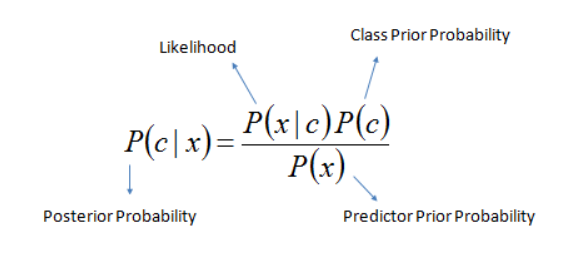Naive_Bayes Formula (Source)

Here, the availability of a registered vehicle, document verification process status, and user interest are considered different parameters when Naive Bayes is used. The primary benefit of using Naive Bayes for a system is its simplicity and accuracy that sometimes even outperforms certain popular classification methods.

Below is a small example of implementing Naive Bayes on the Train dataset using Python that provides the accuracy score derived in the results:

``````# importing required libraries
import pandas as pd
from sklearn.naive_bayes import GaussianNB
from sklearn.metrics import accuracy_score

# read the train and test dataset

# shape of the dataset
print('Shape of training data :',train_data.shape)
print('Shape of testing data :',test_data.shape)

# Now, we need to predict the missing target variable in the test data
# target variable - Survived

# seperate the independent and target variable on training data
train_x = train_data.drop(columns=['Survived'],axis=1)
train_y = train_data['Survived']

# seperate the independent and target variable on testing data
test_x = test_data.drop(columns=['Survived'],axis=1)
test_y = test_data['Survived']

'''
Create the object of the Naive Bayes model
Some parameters are : var_smoothing
Documentation of sklearn GaussianNB:

https://scikit-learn.org/stable/modules/generated/sklearn.naive_bayes.GaussianNB.html

'''
model = GaussianNB()

# fit the model with the training data
model.fit(train_x,train_y)

# predict the target on the train dataset
predict_train = model.predict(train_x)
print('Target on train data',predict_train)

# Accuray Score on train dataset
accuracy_train = accuracy_score(train_y,predict_train)
print('accuracy_score on train dataset : ', accuracy_train)

# predict the target on the test dataset
predict_test = model.predict(test_x)
print('Target on test data',predict_test)

# Accuracy Score on test dataset
accuracy_test = accuracy_score(test_y,predict_test)
print('accuracy_score on test dataset : ', accuracy_test)``````

Apart from these algorithms, there are other popular classification algorithms like SVM (Support Vector Machine) that also provide accurate results for predicting outcomes from given data.

#### 1.2 Regression

A regression algorithm helps in predicting the output in the form of value. For example, if given a dataset of housing pricing, from the input data, these algorithms will be able to provide a fixed price of the house according to the details entered.

##### Linear Regression

Linear regression helps derive the dependency between two variables. Based on the given data, it observes the patterns and later predicts how a change in the value of the independent variable impacts another one. Linear regression helps find out the value rather than classifying or predicting the category. It uses the simple formula of plotting a line on the graph where X-component can help decide the value of Y-component.

The equation of a line is `Y= X.m +b` where m is the slope of the line and b is the intercept. Here, m and b are derived using the method of a sum of the squared difference between data points and the actual regression line. Linear regression can be further expanded in two types: Simple Linear regression where the results depend upon a single variable and Multiple Linear Regression where the results depend upon more than one (two or more) variables.

In simple linear regression, the regression line is plotted in two dimension graphs while for multiple linear regression, the graph gets plotted in the multidimensional arena.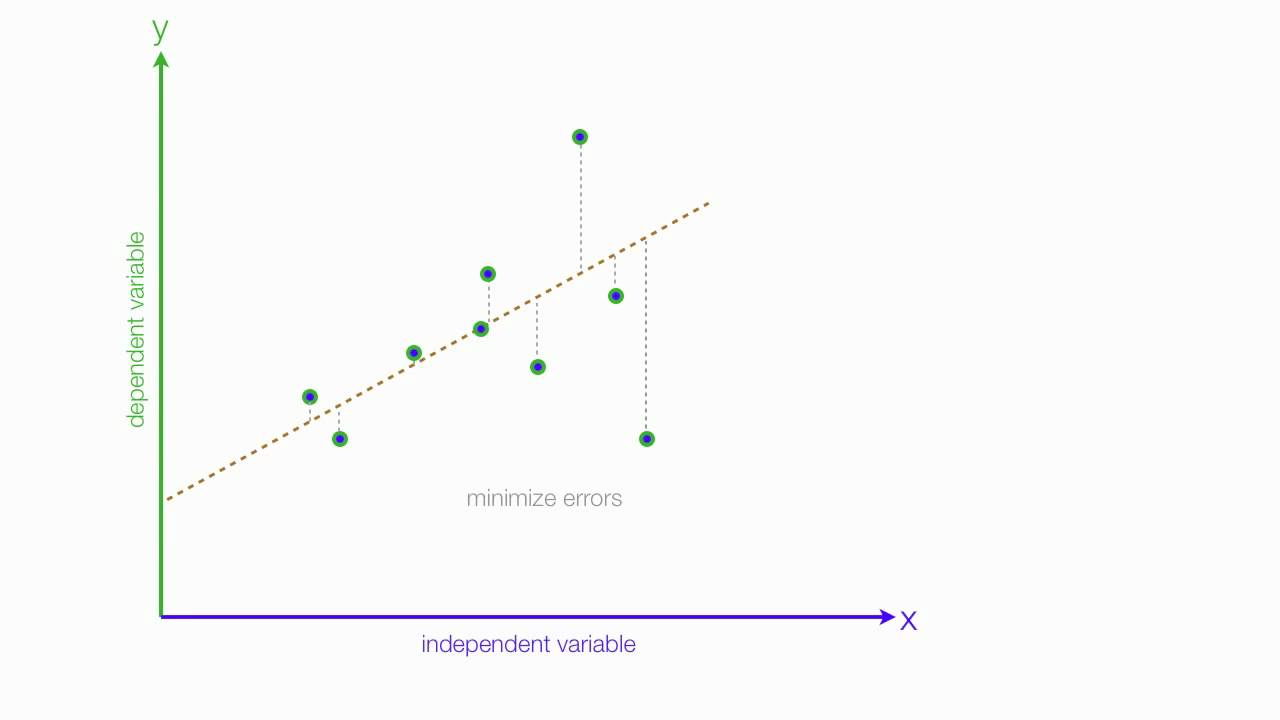Linear Regression (Source)

Below given is a simple implementation of linear regression on Train dataset:

``````# importing required libraries
import pandas as pd
from sklearn.linear_model import LinearRegression
from sklearn.metrics import mean_squared_error

# read the train and test dataset

# shape of the dataset
print('\nShape of training data :',train_data.shape)
print('\nShape of testing data :',test_data.shape)

# Now, we need to predict the missing target variable in the test data
# target variable - Item_Outlet_Sales

# seperate the independent and target variable on training data
train_x = train_data.drop(columns=['Item_Outlet_Sales'],axis=1)
train_y = train_data['Item_Outlet_Sales']

# seperate the independent and target variable on training data
test_x = test_data.drop(columns=['Item_Outlet_Sales'],axis=1)
test_y = test_data['Item_Outlet_Sales']

'''
Create the object of the Linear Regression model
Some parameters are : fit_intercept and normalize
Documentation of sklearn LinearRegression:

https://scikit-learn.org/stable/modules/generated/sklearn.linear_model.LinearRegression.html

'''
model = LinearRegression()

# fit the model with the training data
model.fit(train_x,train_y)

# coefficeints of the trained model
print('\nCoefficient of model :', model.coef_)

# intercept of the model
print('\nIntercept of model',model.intercept_)

# predict the target on the test dataset
predict_train = model.predict(train_x)
print('\nItem_Outlet_Sales on training data',predict_train)

# Root Mean Squared Error on training dataset
rmse_train = mean_squared_error(train_y,predict_train)**(0.5)
print('\nRMSE on train dataset : ', rmse_train)

# predict the target on the testing dataset
predict_test = model.predict(test_x)
print('\nItem_Outlet_Sales on test data',predict_test)

# Root Mean Squared Error on testing dataset
rmse_test = mean_squared_error(test_y,predict_test)**(0.5)
print('\nRMSE on test dataset : ', rmse_test)``````

In supervised learning, the data is already labeled and the algorithms can easily understand what the training sets consist of. However, the true challenge is revealed in unsupervised learning, where the data is not labeled and there are no classes fixed to classify the data, hence in unsupervised learning, clusters and associations are given the highest priority.

### 2. Unsupervised Learning

In unsupervised learning, the data provided to the system is not categorized or labeled and genuinely raw data is fed to the program and the outputs are received according to specified requirements. In a way, unsupervised learning can be taken as the test of AI concepts a program claims to implement. Unsupervised learning focuses on finding relations and establishing patterns among the data and dividing them into clusters or using associations to actually divide the data and provide outputs.

#### 1. Clustering

Clustering is done to identify the entries and put them in clusters where all entries have some common characteristics. K-means clustering algorithm is a popular algorithm that, when executed on the data, divides it into clusters that are formed based on the centroid, which are the data points that are selected. All other values are falling near the centroid point and are divided into clusters and separate clusters are formed. This process of creating centroids and clusters doesn't stop until the value of centroids becomes constant.

The optimum value of clusters can be found, as, after a point, the number of clusters and centroid points slowly begins to decrease, and a particular number is found that is the optimal cluster number.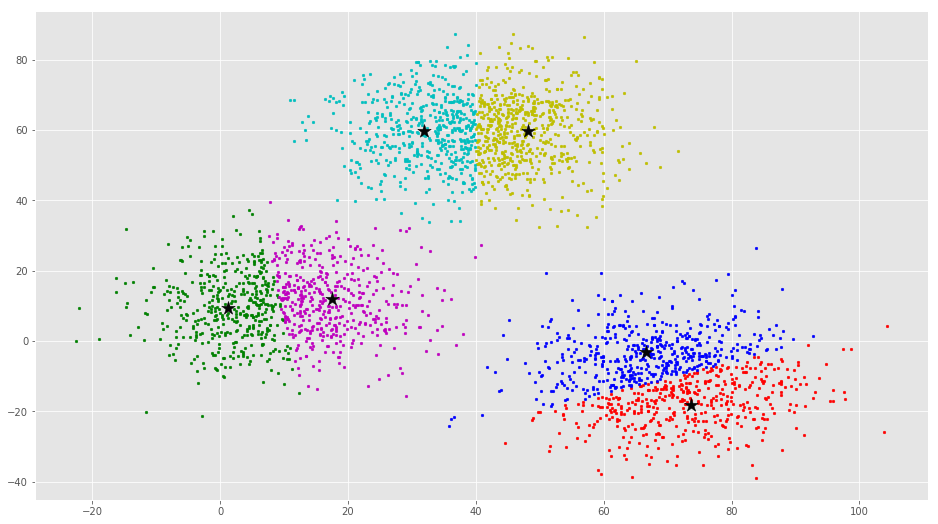K-means Clustering (Source)

Try out the below code to understand how, from the Train dataset, different clusters are formed, as the example provides the total number of clusters created:

``````# importing required libraries
import pandas as pd
from sklearn.cluster import KMeans

# read the train and test dataset

# shape of the dataset
print('Shape of training data :',train_data.shape)
print('Shape of testing data :',test_data.shape)

# Now, we need to divide the training data into differernt clusters
# and predict in which cluster a particular data point belongs.

'''
Create the object of the K-Means model
Some parameters are : n_clusters and max_iter
Documentation of sklearn KMeans:

https://scikit-learn.org/stable/modules/generated/sklearn.cluster.KMeans.html
'''

model = KMeans()

# fit the model with the training data
model.fit(train_data)

# Number of Clusters
print('\nDefault number of Clusters : ',model.n_clusters)

# predict the clusters on the train dataset
predict_train = model.predict(train_data)
print('\nCLusters on train data',predict_train)

# predict the target on the test dataset
predict_test = model.predict(test_data)
print('Clusters on test data',predict_test)

# Now, we will train a model with n_cluster = 3
model_n3 = KMeans(n_clusters=3)

# fit the model with the training data
model_n3.fit(train_data)

# Number of Clusters
print('\nNumber of Clusters : ',model_n3.n_clusters)

# predict the clusters on the train dataset
predict_train_3 = model_n3.predict(train_data)
print('\nCLusters on train data',predict_train_3)

# predict the target on the test dataset
predict_test_3 = model_n3.predict(test_data)
print('Clusters on test data',predict_test_3)``````

#### 2. Association

Association simply works by establishing relationships between the entities and predicts or suggests accordingly. One of the most popular real-world applications of association is the market-basket analysis which is extensively used by Walmart.

Apriori algorithm works wonders when it comes to establishing the relations between items. Especially for retail sectors, this algorithm has achieved tremendous success by increasing their profits. Apriori algorithm aims to establish structural relations between different items. This supports the fundamental reason behind the market-basket analysis and promotes the best practices that help a retail owner know what their users are likely to buy if their shelves arrangements change.

Below is a simple example of implementing the Apriori algorithm on Groceries dataset, and the algorithm predicts what items are likely to be bought together by customers.

``````import numpy as np
import pandas as pd
from mlxtend.frequent_patterns import apriori, association_rules

# Changing the working location to the location of the file
cd C:\Users\Dev\Desktop\Kaggle\Apriori Algorithm

# Exploring the columns of the data
data.columns

# Exploring the different regions of transactions
data.Country.unique()

# Stripping extra spaces in the description
data['Description'] = data['Description'].str.strip()

# Dropping the rows without any invoice number
data.dropna(axis = 0, subset =['InvoiceNo'], inplace = True)
data['InvoiceNo'] = data['InvoiceNo'].astype('str')

# Dropping all transactions which were done on credit
data = data[~data['InvoiceNo'].str.contains('C')]

# Transactions done in France
.groupby(['InvoiceNo', 'Description'])['Quantity']
.sum().unstack().reset_index().fillna(0)
.set_index('InvoiceNo'))

# Defining the hot encoding function to make the data suitable
# for the concerned libraries
def hot_encode(x):
if(x<= 0):
return 0
if(x>= 1):
return 1

# Encoding the datasets

# Building the model
frq_items = apriori(basket_France, min_support = 0.05, use_colnames = True)

# Collecting the inferred rules in a dataframe
rules = association_rules(frq_items, metric ="lift", min_threshold = 1)
rules = rules.sort_values(['confidence', 'lift'], ascending =[False, False])
``````

The outputs generated are the rules derived by calculating the confidence and support values that indicate the likelihood of products bought together by most of the customers.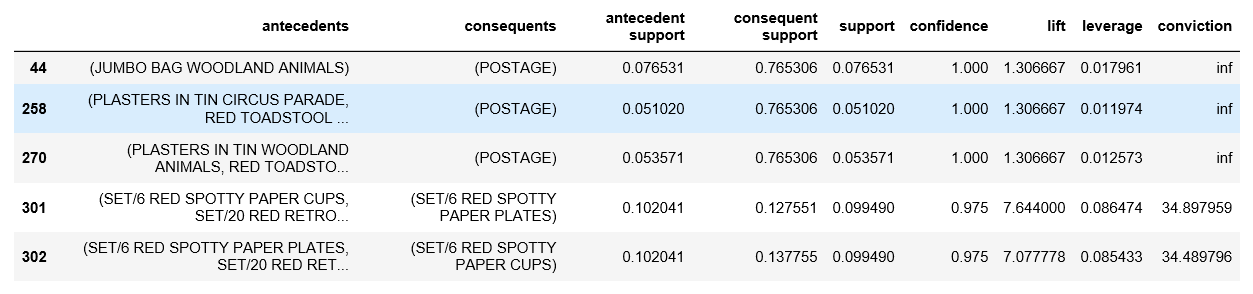Apriori Algorithm Rule Derivation (Source)

### 3. Reinforcement Learning

Reinforcement learning algorithms work on the principle of agent's reactions to the environment. The agent interacts with the given environment and based on the behavior it shows, either the agent is rewarded or it is added to observations. Here, observation is the list of events when the agent is not rewarded and hence, the particular instances are avoided the next time the agent gets to interact with the environment. The most common example of reinforcement learning is dog training.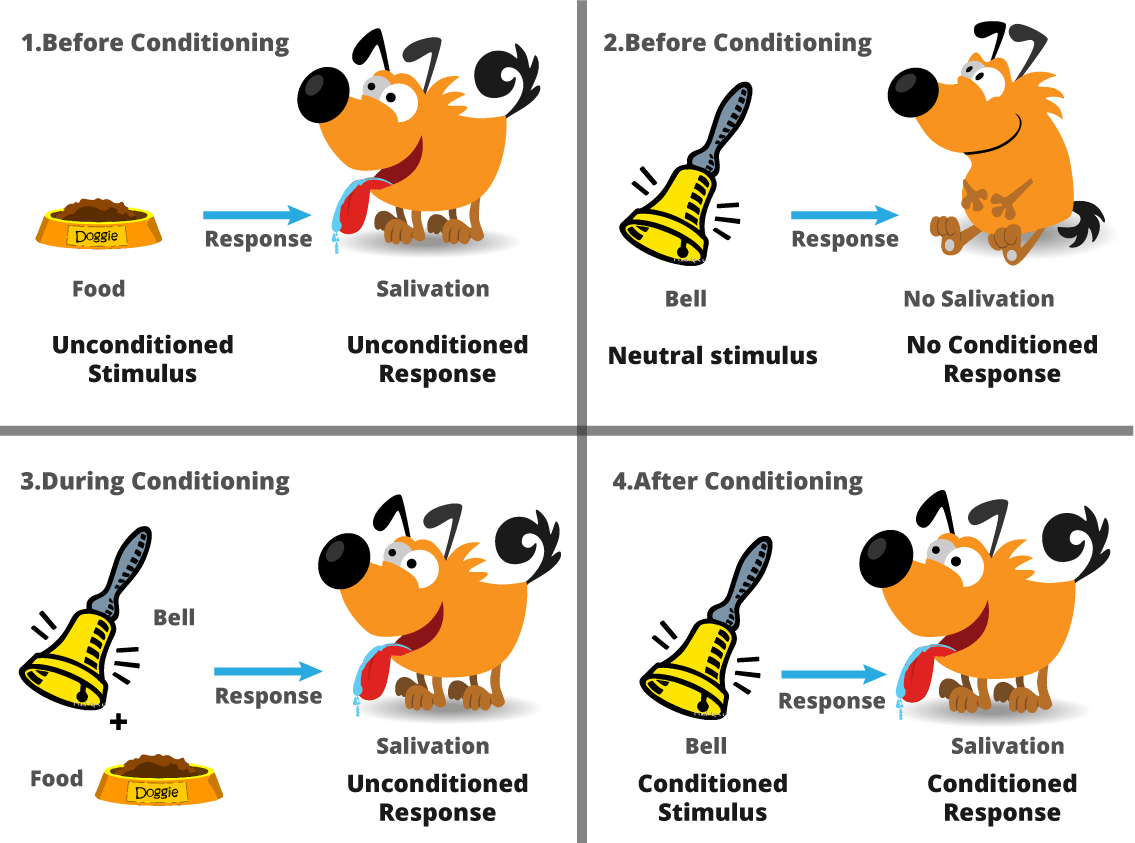Reinforcement Learning (source)

Through reinforcement learning, the agent learns the right ways to interact with the environment and delivers desired results.

## Importance of Choosing the Right Algorithm

As described above, each of these algorithms differs from each other in many ways. For implementing the machine learning algorithms on the datasets available, it is important to understand the nature of data and choose the right algorithm according to the set goals. While the right algorithm can support making smarter solutions, the algorithm lacking the functionalities can impact the entire intelligence system badly and may provide wrong outputs.

To choose the right machine learning algorithm, seeking support from expert data scientists is advisable to know which can fit in the requirements and provide accurate results. As the inventions are taking the concepts of machine learning and artificial intelligence many steps further, data science promises a bright career in the future for every machine learning enthusiast.

Machine Learning: An Introduction

Artificial Intelligence: Machine Learning and Predictive Analytics

Machine Learning

Topics:
machine learning, data science, ml, artificial intelligence, supervised learning, unsupervised learning, decision trees, ml algorithms

Opinions expressed by DZone contributors are their own.